# Posts published in “Recursion”

Alex and Lee continue their games with piles of stones.  There are a number of piles arranged in a row, and each pile has a positive integer number of stones piles[i].  The objective of the game is to end with the most stones.

Alex and Lee take turns, with Alex starting first.  Initially, M = 1.

On each player’s turn, that player can take all the stones in the first X remaining piles, where 1 <= X <= 2M.  Then, we set M = max(M, X).

The game continues until all the stones have been taken.

Assuming Alex and Lee play optimally, return the maximum number of stones Alex can get.

Example 1:

Input: piles = [2,7,9,4,4]
Output: 10
Explanation:  If Alex takes one pile at the beginning, Lee takes two piles, then Alex takes 2 piles again. Alex can get 2 + 4 + 4 = 10 piles in total. If Alex takes two piles at the beginning, then Lee can take all three piles left. In this case, Alex get 2 + 7 = 9 piles in total. So we return 10 since it's larger.


Constraints:

• 1 <= piles.length <= 100
• 1 <= piles[i] <= 10 ^ 4

## Solution: Recursion + Memoization

def solve(s, m) = max diff score between two players starting from s for the given M.

cache[s][M] = max{sum(piles[s:s+x]) – solve(s+x, max(x, M)}, 1 <= x <= 2*M, s + x <= n

Time complexity: O(n^3)
Space complexity: O(n^2)

## C++

Return the result of evaluating a given boolean expression, represented as a string.

An expression can either be:

• "t", evaluating to True;
• "f", evaluating to False;
• "!(expr)", evaluating to the logical NOT of the inner expression expr;
• "&(expr1,expr2,...)", evaluating to the logical AND of 2 or more inner expressions expr1, expr2, ...;
• "|(expr1,expr2,...)", evaluating to the logical OR of 2 or more inner expressions expr1, expr2, ...

Example 1:

Input: expression = "!(f)"
Output: true


Example 2:

Input: expression = "|(f,t)"
Output: true


Example 3:

Input: expression = "&(t,f)"
Output: false


Example 4:

Input: expression = "|(&(t,f,t),!(t))"
Output: false

## Solution: Recursion

Time complexity: O(n)
Space complexity: O(n)

## Java

Given an encoded string, return it’s decoded string.

The encoding rule is: k[encoded_string], where the encoded_string inside the square brackets is being repeated exactly k times. Note that k is guaranteed to be a positive integer.

You may assume that the input string is always valid; No extra white spaces, square brackets are well-formed, etc.

Furthermore, you may assume that the original data does not contain any digits and that digits are only for those repeat numbers, k. For example, there won’t be input like 3a or 2.

Examples:

s = "3[a]2[bc]", return "aaabcbc".
s = "3[a2[c]]", return "accaccacc".
s = "2[abc]3[cd]ef", return "abcabccdcdcdef".

## Solution 1: Recursion

Time complexity: O(n^2)
Space complexity: O(n)

## C++

In the “100 game,” two players take turns adding, to a running total, any integer from 1..10. The player who first causes the running total to reach or exceed 100 wins.

What if we change the game so that players cannot re-use integers?

For example, two players might take turns drawing from a common pool of numbers of 1..15 without replacement until they reach a total >= 100.

Given an integer maxChoosableInteger and another integer desiredTotal, determine if the first player to move can force a win, assuming both players play optimally.

You can always assume that maxChoosableInteger will not be larger than 20 and desiredTotal will not be larger than 300.

Example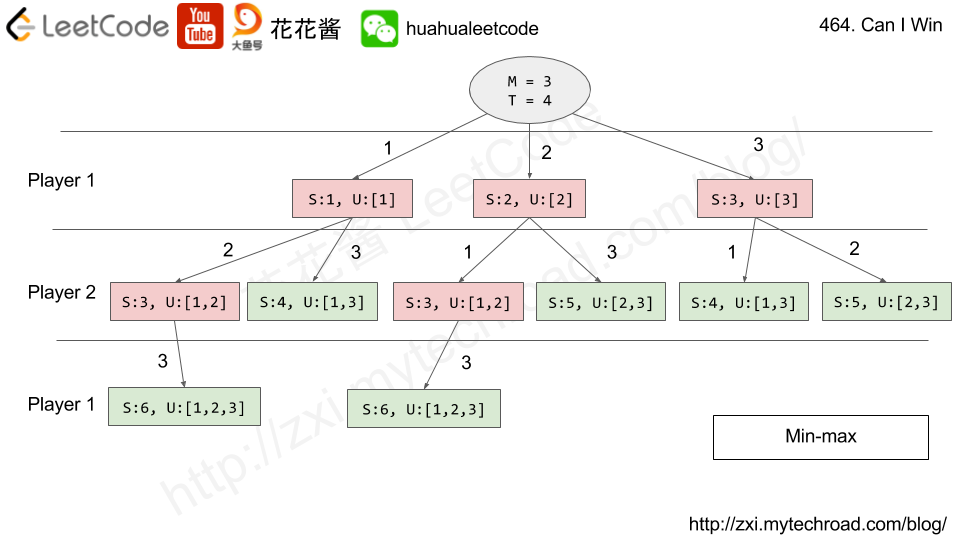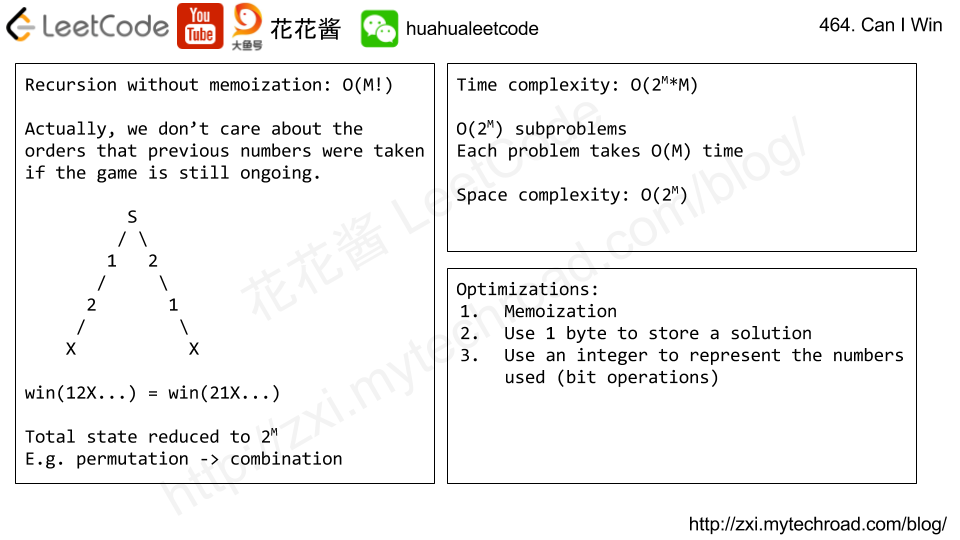Solution: Recursion with memoization

Time complexity: O(2^M)

Space complexity: O(2^M)

C++

Java

Python3

Problem:

You are given a string expression representing a Lisp-like expression to return the integer value of.

The syntax for these expressions is given as follows.

• An expression is either an integer, a let-expression, an add-expression, a mult-expression, or an assigned variable. Expressions always evaluate to a single integer.
• (An integer could be positive or negative.)
• A let-expression takes the form (let v1 e1 v2 e2 ... vn en expr), where let is always the string "let", then there are 1 or more pairs of alternating variables and expressions, meaning that the first variable v1is assigned the value of the expression e1, the second variable v2 is assigned the value of the expression e2, and so on sequentially; and then the value of this let-expression is the value of the expression expr.
• An add-expression takes the form (add e1 e2) where add is always the string "add", there are always two expressions e1, e2, and this expression evaluates to the addition of the evaluation of e1 and the evaluation of e2.
• A mult-expression takes the form (mult e1 e2) where mult is always the string "mult", there are always two expressions e1, e2, and this expression evaluates to the multiplication of the evaluation of e1 and the evaluation of e2.
• For the purposes of this question, we will use a smaller subset of variable names. A variable starts with a lowercase letter, then zero or more lowercase letters or digits. Additionally for your convenience, the names “add”, “let”, or “mult” are protected and will never be used as variable names.
• Finally, there is the concept of scope. When an expression of a variable name is evaluated, within the context of that evaluation, the innermost scope (in terms of parentheses) is checked first for the value of that variable, and then outer scopes are checked sequentially. It is guaranteed that every expression is legal. Please see the examples for more details on scope.

Evaluation Examples:

Note:

• The given string expression is well formatted: There are no leading or trailing spaces, there is only a single space separating different components of the string, and no space between adjacent parentheses. The expression is guaranteed to be legal and evaluate to an integer.
• The length of expression is at most 2000. (It is also non-empty, as that would not be a legal expression.)
• The answer and all intermediate calculations of that answer are guaranteed to fit in a 32-bit integer.

Idea:

Recursive parsing

Time complexity: O(n^2) in worst case O(n) in practice

Space complexity: O(n)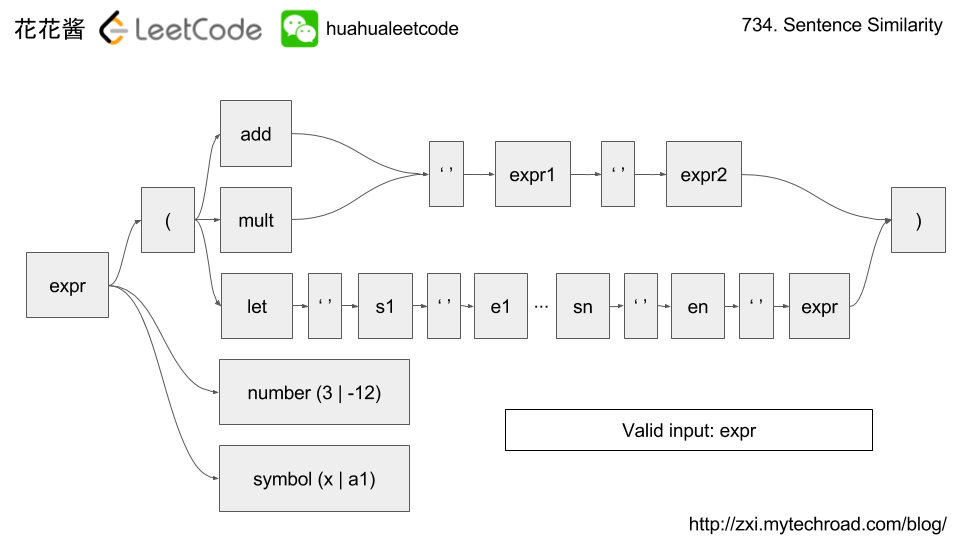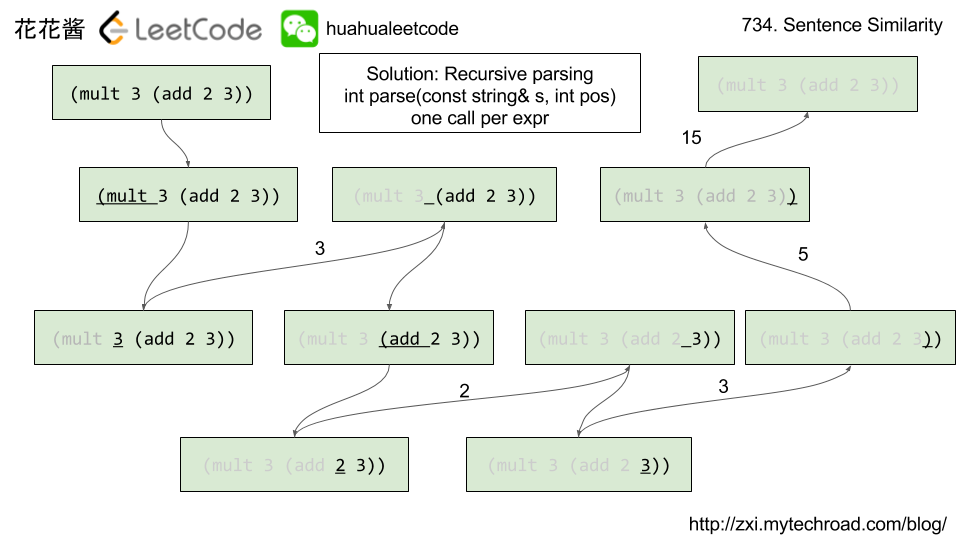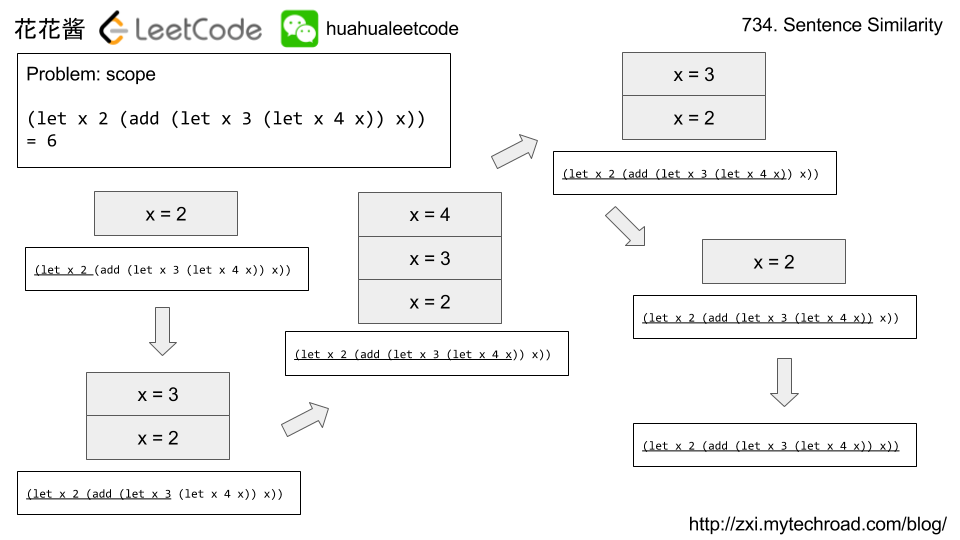Mission News Theme by Compete Themes.